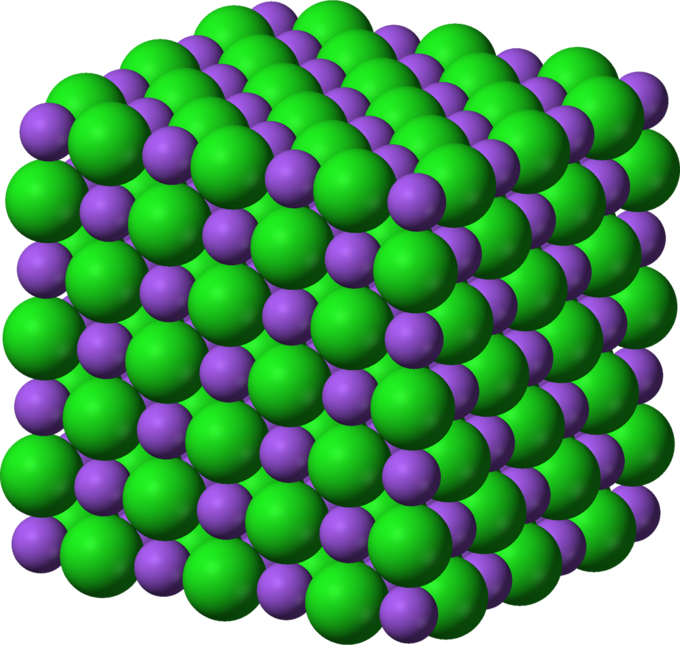## Lattice Energy

#### Learning Objective

• Describe lattice energy and the factors that affect it

#### Key Points

• Lattice energy is defined as the energy required to separate a mole of an ionic solid into gaseous ions.
• Lattice energy cannot be measured empirically, but it can be calculated using electrostatics or estimated using the Born-Haber cycle.
• Two main factors that contribute to the magnitude of the lattice energy are the charge and radius of the bonded ions.

#### Terms

• exothermic reactionA process which releases heat into its surroundings.
• lattice energyThe amount of energy released upon formation of a crystalline ionic solid from gaseous ions.

## Definition of Lattice Energy

Lattice energy is an estimate of the bond strength in ionic compounds. It is defined as the heat of formation for ions of opposite charge in the gas phase to combine into an ionic solid. As an example, the lattice energy of sodium chloride, NaCl, is the energy released when gaseous Na+ and Cl ions come together to form a lattice of alternating ions in the NaCl crystal.

$Na^+ (g) + Cl^- (g) \rightarrow NaCl (s) \;\;\;\;\;\;\;\;\;\;\;\;\;\;\; \Delta H=-787.3 kJ/mol$

The negative sign of the energy is indicative of an exothermic reaction.

Alternatively, lattice energy can be thought of as the energy required to separate a mole of an ionic solid into the gaseous form of its ions (that is, the reverse of the reaction shown above).NaCl Crystalline LatticeSodium ions (Na+) and chloride(Cl–) ions, depicted in purple and green respectively, alternate in the crystal lattice of solid NaCl.

Lattice energy cannot be determined experimentally due to the difficulty in isolating gaseous ions. The energy value can be estimated using the Born-Haber cycle, or it can be calculated theoretically with an electrostatic examination of the crystal structure.

## Factors Affecting Lattice Energy

In 1918, Born and Lande presented the following model for lattice energy:

$E = - \frac {N_AMz^+z^-e^2}{4 \pi \epsilon_o r_o} (1-\frac {1}{n})$

In this equation, NA is Avogadro’s constant; M is the Madelung constant, which depends on the crystal geometry; z+ is the charge number of the cation; z is the charge number of the anion; e is the elementary charge of the electron; n is the Born exponent, a characteristic of the compressibility of the solid; $\epsilon _o$ is the permittivity of free space; and r0 is the distance to the closest ion.

This model emphasizes two main factors that contribute to the lattice energy of an ionic solid: the charge on the ions, and the radius, or size, of the ions. The effect of those factors is:

• as the charge of the ions increases, the lattice energy increases
• as the size of the ions increases, the lattice energy decreases

Lattice energies are also important in predicting the solubility of ionic solids in H2O. Ionic compounds with smaller lattice energies tend to be more soluble in H2O.